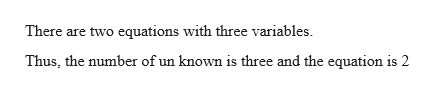# Explain why a system of two equations with three variables cannot have exactly one ordered triple as its solution.

Question
1 views

Explain why a system of two equations with three variables cannot have exactly one ordered triple as its solution.

check_circle

Step 1help_outlineImage TranscriptioncloseThere are two equations with three variables. Thus, the number of un known is three and the equation is 2 fullscreen

### Want to see the full answer?

See Solution

#### Want to see this answer and more?

Solutions are written by subject experts who are available 24/7. Questions are typically answered within 1 hour.*

See Solution
*Response times may vary by subject and question.
Tagged in

### Equations and In-equations